# 9-3 Lennard-Jones potential

Jump to: navigation, search

The 9-3 Lennard-Jones potential is related to the Lennard-Jones potential. It has the following form: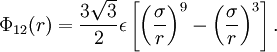$\Phi_{12}(r) = \frac{ 3 \sqrt{3}}{ 2} \epsilon \left[ \left( \frac{\sigma}{r} \right)^9 - \left( \frac{ \sigma }{r} \right)^3 \right].$

where$\Phi_{12}(r)$ is the intermolecular pair potential and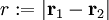$r := |\mathbf{r}_1 - \mathbf{r}_2|$. The minimum value of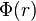$\Phi(r)$ is obtained at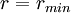$r = r_{min}$, with

•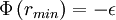$\Phi \left( r_{min} \right) = - \epsilon$,
•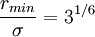$\frac{ r_{min} }{\sigma} = 3^{1/6}$

## Applications

It is commonly used to model the interaction between the particles of a fluid with a flat structureless solid wall or vice versa (Ref. 1).

## Interaction between a solid and a fluid molecule

Let us consider the space divided in two regions:

•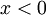$x < 0$: this region is occupied by a diffuse solid with density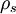$\rho_s$ composed of 12-6 Lennard-Jones atoms

with parameters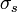$\sigma_s$ and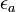$\epsilon_a$

Our aim is to compute the total interaction between this solid and a molecule located at a position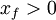$x_f > 0$. Such an interaction can be computed using cylindrical coordinates.

The interaction will be: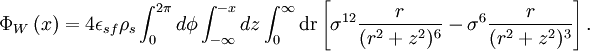$\Phi_{W} \left( x \right) = 4 \epsilon_{sf} \rho_{s} \int_{0}^{2\pi} d \phi \int_{-\infty}^{-x} d z \int_{0}^{\infty} \textrm{d r} \left[ \sigma^{12} \frac{ r} {(r^2 + z^2)^{6}} - \sigma^6 \frac{r}{(r^2 + z^2 )^{3} }\right] .$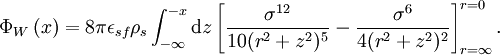$\Phi_{W} \left( x \right) = 8 \pi \epsilon_{sf} \rho_{s} \int_{-\infty}^{-x} {\textrm d z} \left[ \frac{ \sigma^{12}} { 10 (r^2 + z^2)^5} - \frac{\sigma^6 }{ 4 (r^2 + z^2 )^{2} }\right]^{r=0}_{r=\infty} .$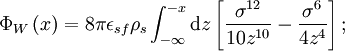$\Phi_{W} \left( x \right) = 8 \pi \epsilon_{sf} \rho_{s} \int_{-\infty}^{-x} {\textrm d z} \left[ \frac{ \sigma^{12}} { 10 z^{10} } - \frac{\sigma^6 }{ 4 z^4 } \right];$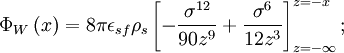$\Phi_{W} \left( x \right) = 8 \pi \epsilon_{sf} \rho_s \left[ - \frac{ \sigma^{12}} { 90 z^{9} } + \frac{\sigma^6 }{ 12 z^3 } \right]_{z=-\infty}^{z=-x};$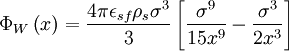$\Phi_{W} \left( x \right) = \frac{4 \pi \epsilon_{sf} \rho_s \sigma^3}{3} \left[ \frac{ \sigma^{9}} { 15 x^{9} } - \frac{\sigma^3 }{ 2 x^3 } \right]$